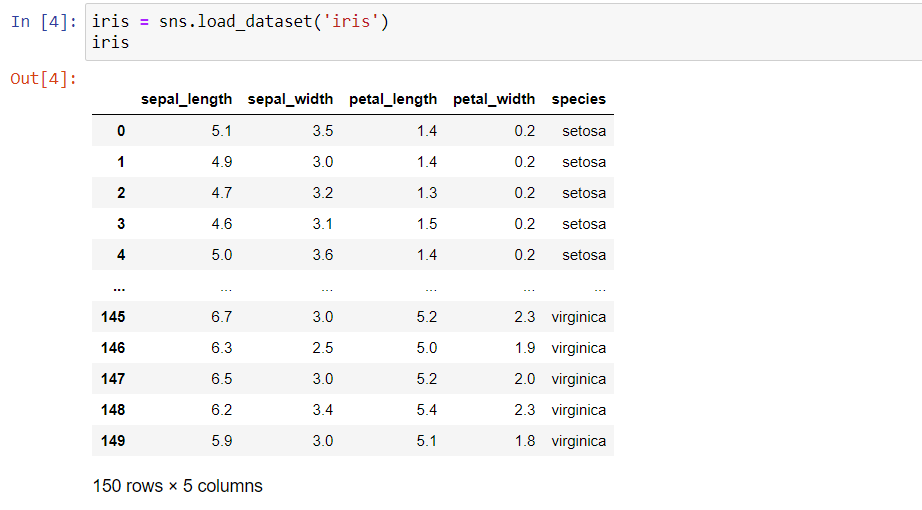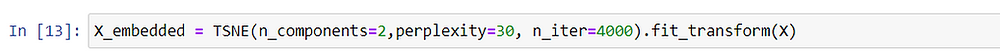Search

# Dimensionality reduction using t-SNE

Written Abhinav T K

## Introduction

The number of features in a dataset is called its dimensionality. Dimensionality reduction is the technique of reducing the number of dimensions.

Need for dimensionality reduction:

A large number of features in a dataset can cause our Machine Learning model to perform poorly. As we increase the number of features, the need for data points increases in an exponential order for our ML model to work well. This is called the ‘curse of dimensionality’.

Reducing the dimensions to two or three can also help to visualize the data and gain meaningful insights from it.

Here I’m going to discuss t-SNE which is a popular dimensionality reduction technique.

## t-distributed Stochastic Neighbor Embedding

t-SNE is used to represent high dimensional data to a low dimensional space without losing much of its information. It is a powerful tool to visualize high dimensional data.

## How t-SNE works?

t-SNE transforms a high dimensional data to a low dimensional space where similar samples are grouped together and dissimilar samples are at a distant point. t-SNE picks up points in the high dimensional space which are in the close neighbourhood and place it in the low dimensional space. The nearby samples are called similar samples. t-SNE converts the similarity between data points to joint probabilities and tries to minimize the Kullback-Leibler divergence between the joint probabilities of the high-dimensional space and the low dimensional embedding. So the points with high joint probabilities will be clustered together in the low dimensional space.

## What does the full form of t-SNE which sounds highly technical mean?

t- distribution is the distribution of the joint probabilities of low dimensional space points. It is similar to normal distribution because of its bell shape but with heavier tails. Without t-distribution, the clusters have a chance of clumping together and it will be harder to differentiate.

Neighbour embedding means placing the nearby points from the high dimensional space to the low dimensional space.## Hyperparameters of t-SNE:

Perplexity

Perplexity is the number of nearest neighbours of each point in the high dimensional space whose geometric distance is preserved while embedding to lower-dimensional space. Typical values of perplexity range from 5 to 50. As a rule of thumb with a larger dataset, the value of perplexity should be increased. This is a tricky hyperparameter since different values can lead to significantly different results.

Number of iterations

t-SNE is an iterative algorithm. After each iteration, it tries to improve the embedding of nearest neighbours as possible. Ideally more the number of iterations, the better. But it may not be feasible for large datasets because it can take a lot of time to run. On the other hand, a small number of iterations would result in a meaningless t-SNE plot where all the points are clumped together. In scikit-learn, the default number of iterations is 1000.

Best way to tune the hyperparameters is by hit and trial, that is by checking what works and what doesn’t.

## Applying t-SNE on Iris dataset

Iris dataset consists of 50 samples from each of three species of Iris (Iris setosa, Iris virginica and Iris versicolor). From each sample, four features were measured: the length and the width of the sepals and petals, in centimetres.

Let’s apply t-SNE on this dataset using scikit learn.

1. Import the required libraries2. We can load the dataset using the seaborn library.The dataset contains 150 rows and 5 columns. The ‘species’ column tells to which species each sample belongs to.

3. Let’s make Numpy arrays X with all the features and Y for the species column.Now let’s apply t-SNE on X using the TSNE class from sklearn.manifold. Here we have taken perplexity = 30, n_components = 2 and n_iter = 4000. n_components is the number of components of the low dimensional space.The method fit_transform will return a numpy array X_embedded of shape 150x2 (no. of samples, n_components).

4. Now let's plot X_embedded to see how t-SNE performed on our data.Inferences from the plot:

• We can see that t-SNE has done a good job in forming different clusters of our data according to different iris species.

• We can improve the plot even more by changing the perplexity and number of iterations accordingly.

About the person behind the keyboard: Abhinav is pursuing B.tech from IIT Hyderabad and is a passionate engineer. If you guys want to contact him, just click on his name.